# Parity of modular operations

2009-11-29, last updated 2014-03-03

For a problem I am trying to solve I want to find out if I can quickly calculate the odd/even parity of an inverse modulo a large prime. That is, I want to know:

$\left( a^{m-2} \mod m \right) \mod 2$

Where $a$ is typically less than a hundred thousand and $m$ is a 64 bit prime.

## Parity of modular multiplication

Let’s first investigate the related question of the parity of the modular product:

$\left( a \cdot b \mod m \right) \mod 2$

Now, if $a$ or $b$ is zero then the parity will also be zero. The other case is however more interesting. Lets plot the parity for a few small primes $m$.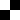,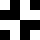,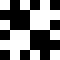,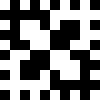,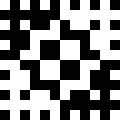,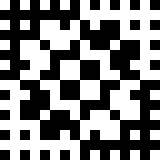Now a few things can be noticed, first that the whole thing is symmetric over the diagonal, a consequence of the commutativity of multiplication. Second, there are two anti-symmetries: the left half is the exact opposite of the right and the top half is the exact opposite of the bottom half. This leads to the relation:

\begin{aligned} &\left(a \cdot b \mod m \right) \\ &\equiv \left( (m - a) \cdot b + 1\mod m \right)\mod 2 \\ &\equiv \left(- a \cdot b + 1 \mod m \right)\mod 2 \end{aligned}

The explanation is that a negative number gets subtracted from m, which is odd, such that the result has its parity flipped.

Another peculiarity in the pattern is that there seems to be some kind of grid xor-ed with the image. This grid corresponds to a $\cdot b \mod 2$ and can be xored out: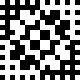$\bigoplus$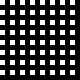$=$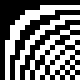The pattern that remains consists of hyperbolas corresponding to the number of $m$’s that need to be subtracted to compute the $\mod m$. Since $m$ is odd each additional subtraction results in a parity flip.

The top left half of the hyperbola pattern appears reasonably well behaved, the rest appears distorted due to aliasing, but that is not a problem, since these can be calculated using the anti-symmetries. I verified that the top-left quarter stays well behaved for a few large primes $m$.

Unfortunately, I can’t see a faster way to calculate hyperbolas than to perform an division, so speeding up the multiplication seems to be a dead end.

## Parity of modular exponentiation

Next attempt, maybe some structure emerges in the exponentiation process. So let’s investigate

$\left( a ^ b \mod m \right) \mod 2$

Again, first the small primes: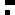,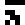,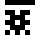,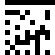,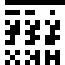,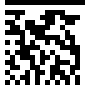It’s seems almost, but not entirely, random, like a cellular automaton or a broken pseudo random generator. Especially the next-to-last one, $a^b \mod 13$ seems to have a repeating pattern in it. Investigating some larger primes for $m$ I stumbled upon 281, which has the following pattern:

There is clearly a grid of white lines going on in this pattern. To investigate this further I took the discrete cosine transform:

A pattern of vertical lines emerges, which means that there should be a lot of correlation between the columns of the pattern.

Now lets take a prime number that does not have a white line grid, such as the next number, 283. It’s pattern is:

This pattern does seem completely random, however, when we apply the cosine transform the vertical lines re-appear, although less pronounced:

This seems to happen for every prime I try, and I can’t currently explain where it comes from. It seems that the length of the pattern is a divisor of $\varphi(m)$ and thus related to Fermat’s little theorem and Euler’s theorem, I should study this further sometime, but for now it doesn’t seem likely that this will result in faster computation of modular exponentiation parities.